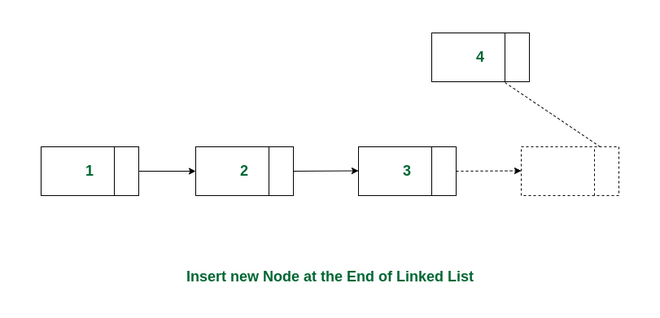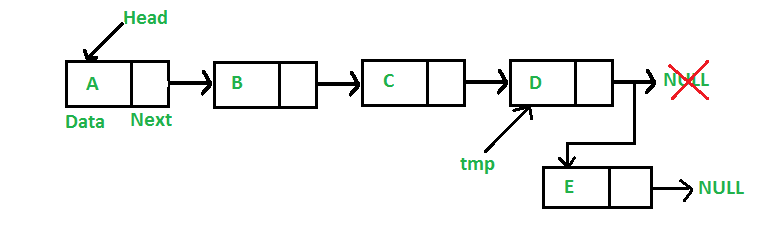GeeksforGeeks App
Open AppBrowser
Continue

# Insert Node at the End of a Linked List

Given a linked list, the task is to insert a new node at the end of the linked list.Insert a node at the end of a linked list

Example:

Input: LinkedList = 2->3->4->5, NewNode = 1

Input: LinkedList = , NewNode = 1

Approach:

To insert a node at the end of a Linked List, we need to:

• Go to the last node of the Linked List
• Change the next pointer of last node from NULL to the new node
• Make the next pointer of new node as NULL to show the end of Linked ListFollowing is the approach to add a new node at the end of the linked list:

• Create a new node
• Store the head reference in a temporary variable
• Set the next pointer of the new node as NULL since it will be the last node
• If the Linked List is empty, make the new node as the head and return
• Else traverse till the last node
• Change the next pointer of the last node to point to the new node

Below is the implementation of the approach:

## C++

```// C++ program to demonstrate inserting a node
// at the end of a Linked List
#include <bits/stdc++.h>
using namespace std;

class Node {
public:
int data;
Node* next;
};

// Given a reference (pointer to pointer)
// to the head of a list and an int, inserts
// a new node at the front of the list.
{
// Create a new node
Node* new_node = new Node();
new_node->data = new_data;

// Make the new node point to the current head

// Update the head to point to the new node
}

// Given a reference (pointer to pointer)
// to the head of a list and an int,
// appends a new node at the end
{
// Create a new node
Node* new_node = new Node();
new_node->data = new_data;

// Store the head reference in a temporary variable

// Set the next pointer of the new node as NULL since it
// will be the last node
new_node->next = NULL;

// If the Linked List is empty, make the new node as the
return;
}

// Else traverse till the last node
while (last->next != NULL) {
last = last->next;
}

// Change the next pointer of the last node to point to
// the new node
last->next = new_node;
}

// This function prints the contents of
void printList(Node* node)
{
while (node != NULL) {
cout << " " << node->data;
node = node->next;
}
}

// Driver code
int main()
{

// Insert nodes at the beginning of the linked list

cout << "Created Linked list is: ";

// Insert 1 at the end

cout << "\nAfter inserting 1 at the end: ";

return 0;
}```

## C

```#include <stdio.h>
#include <stdlib.h>

struct Node {
int data;
struct Node* next;
};

// Given a reference (pointer to pointer)
// to the head of a list and an int, inserts
// a new node on the front of the list.
void push(struct Node** head_ref, int new_data)
{
// Create a new node
struct Node* new_node
= (struct Node*)malloc(sizeof(struct Node));
new_node->data = new_data;

// Make the new node point to the current head

// Update the head to point to the new node
}

// Given a reference (pointer to pointer)
// to the head of a list and an int,
// appends a new node at the end
void append(struct Node** head_ref, int new_data)
{
// Create a new node
struct Node* new_node
= (struct Node*)malloc(sizeof(struct Node));
new_node->data = new_data;

// Store the head reference in a temporary variable

// Set the next pointer of the new node as NULL since it
// will be the last node
new_node->next = NULL;

// If the Linked List is empty, make the new node as the
return;
}

// Else traverse till the last node
while (last->next != NULL) {
last = last->next;
}

// Change the next pointer of the last node to point to
// the new node
last->next = new_node;
}

// This function prints the contents of
void printList(struct Node* node)
{
while (node != NULL) {
printf(" %d", node->data);
node = node->next;
}
}

// Driver code
int main()
{

// Insert nodes at the beginning of the linked list

// Insert 1 at the end

printf("\nAfter inserting 1 at the end: ");

return 0;
}```

## Java

```class Node {
int data;
Node next;

Node(int data)
{
this.data = data;
next = null;
}
}

// Inserts a new node at the front of the list
void push(int new_data)
{
Node new_node = new Node(new_data);
}

// Appends a new node at the end of the list
void append(int new_data)
{
Node new_node = new Node(new_data);

return;
}

while (last.next != null) {
last = last.next;
}

last.next = new_node;
}

// Prints the contents of the linked list
void printList()
{
while (node != null) {
System.out.print(node.data + " ");
node = node.next;
}
}
}

public class Main {
public static void main(String[] args)
{

// Insert nodes at the beginning of the linked list

// Insert 1 at the end

System.out.print(
"\nAfter inserting 1 at the end: ");
}
}```

## Python3

```# A linked list node
class Node:
def __init__(self, data):
self.data = data
self.next = None

# Given a reference (pointer to pointer)
# to the head of a list and an int, inserts
# a new node on the front of the list.

# Create a new node
new_node = Node(new_data)

# Make the new node point to the current head

# Update the head to point to the new node
return new_node

# Given a reference (pointer to pointer)
# to the head of a list and an int,
# appends a new node at the end

# Create a new node
new_node = Node(new_data)

# Store the head reference in a temporary variable

# Set the next pointer of the new node as None since it
# will be the last node
new_node.next = None

# If the Linked List is empty, make the new node as the
return new_node

# Else traverse till the last node
while last.next is not None:
last = last.next

# Change the next pointer of the last node to point to
# the new node
last.next = new_node

# This function prints the contents of

def printList(node):
while node is not None:
print(node.data, end=" ")
node = node.next

# Driver code
if __name__ == "__main__":

# Insert nodes at the beginning of the linked list

# Insert 1 at the end

print("\nAfter inserting 1 at the end:")
```

## C#

```using System;

public class Node {
public int data;
public Node next;
}

// Given a reference (pointer to pointer)
// to the head of a list and an int, inserts
// a new node on the front of the list.
public static void Push(ref Node head_ref, int new_data)
{
// Create a new node
Node new_node = new Node();
new_node.data = new_data;

// Make the new node point to the current head

// Update the head to point to the new node
}

// Given a reference (pointer to pointer)
// to the head of a list and an int,
// appends a new node at the end
public static void Append(ref Node head_ref,
int new_data)
{
// Create a new node
Node new_node = new Node();
new_node.data = new_data;

// If the Linked List is empty, make the new node as
return;
}

// Else traverse till the last node
while (last.next != null) {
last = last.next;
}

// Change the next pointer of the last node to point
// to the new node
last.next = new_node;
}

// This function prints the contents of
public static void PrintList(Node node)
{
while (node != null) {
Console.Write(" " + node.data);
node = node.next;
}
}

// Driver code
public static void Main(string[] args)
{

// Insert nodes at the beginning of the linked list

// Insert 1 at the end

Console.Write("\nAfter inserting 1 at the end:");
```Created Linked list is:  2 3 4 5 6